# Electricity and Energy

## Electricity and Energy

Congratulations - you have completed Electricity and Energy. You scored %%SCORE%% out of %%TOTAL%%. Your performance has been rated as %%RATING%%
 Question 1
Electric current is defined as the rate of flow of________
 A energy B power C mass D charges
 Question 2
Electric charge is expressed in ________
 A Volt B Joule C Coulomb D Ohm
 Question 3
The unit of electric current is ______
 A ampere B volt C watt D kilo-watt
 Question 4
Which instrument is used to measure current?
 A voltmeter B ammeter C galvanometer D ohmmeter
 Question 5
The amount of work done to move a unit charge from one point to the other is _______
 A resistance B current C potential D none of the above
 Question 6
Which quantity is measures by voltmeter?
 A current B potential C resistance D capacitance
 Question 7
Ohm’s law gives the relation between potential difference and ______
 A emf B temperature C resistance D current
 Question 8
In an electrical circuit, voltmeter reads 24V and ammeter reads 6A. the value of resistance is _________
 A 40Ω B 2 Ω C 0.25 Ω D 4 Ω
 Question 9
Ohm is also expressed as _________
 A volt/ampere B volt/coulomb C newton D none
 Question 10
Which property of a body oppose the flow of electric charge through it?
 A potential B resistance C temperature D none
 Question 11
When more than one resistors are in series, which quantity remains the term?
 A Current B Potential difference C Resistance D Power
 Question 12
To increase resistance, one should use combination of resistors in _______
 A series B parallel C series and parallel D series always
 Question 13
Which quantity remains the same when resistors are connected in parallel?
 A current B potential difference C emf D resistance
 Question 14
1 Joule / 1 coulomb______
 A 1 watt B 1 kwh C 1 volt D 1 ampere
 Question 15
When a resistance of 6Ω is connected with a cell of electromotive force 1.5V, the current flowing through the cell is ________
 A 2A B 0.25A C 0.5A D 9A
 Question 16
Three resistances 2 Ω, 2 Ω and 2 Ω are connected in parallel with each other. The effective resistance is _______
 A 1.5 Ω B 0.66 Ω C 6 Ω D 0.75 Ω
 Question 17
The value of current flowing through a circuit consisting of two resistances 6 Ω and 18 Ω in series with a battery of 3 Ω is______
 A 0.5A B 0.125A C 6A D 0.25A
 Question 18
Which of the following is used to measure potential difference?
 A ammeter B galvanometer C voltmeter D wattmeter
 Question 19
The work done in moving a charge of 10c through two points having potential difference of 5V is________
 A 100J B 50J C 5J D 250J
 Question 20
The commonly used safely fuse wire is made of_______
 A nickel B lead C an alloy of tin and lead D copper
 Question 21
According to international convention of colour coding in a wire______
 A live is red, neutral is black B live is red, neutral is green C live is brown, neutral is blue D live is green, neutral is black
 Question 22
Kilowatt-hour is the unit of _______
 A potential difference B electric power C electrical energy D charge
 Question 23
Name the physical quantity which is measured in KW____
 A electric energy B electric power C electric current D electric potential
 Question 24
Negatively and positively charged ions which are free to move constitute a current___________
 A In conductors B In an electrolytes C In metals D In liquids
 Question 25
Who built the first battery?
 A Lechlanche B Daniel C Volta D Edison
 Question 26
In cells, chemical energy is converted into ______
 A mechanical energy B heat energy C sound energy D electrical energy
 Question 27
The emf generated in voltaic cell is ________
 A 1.5 V B 2V C 1.08V D 2.2V
 Question 28
In ________ positively and negatively charged ions that are free to move constitute an electric current.
 A conductors B metals C liquids D electrolyte
 Question 29
The emf 1.5V is produced by _____
 A Daniel cell B Voltaic cell C Lechlanche D Lead acid accumulator
 Question 30
Which of the following is not a primary cell?
 A Daniel cell B Lechlanche C Lead acid accumulator D none
 Question 31
Which of these is not a feature of Daniel cell?
 A Copper vessel B Zinc rod C Copper sulphate solution D Carbon rod
 Question 32
Charging is a process of reproducing ________
 A active materials B current C voltage D power
 Question 33
The emf obtained from secondary cell is ______
 A 1.08V B 1.5V C 2.2 V D 1.2 V
 Question 34
A good fuel should _______
 A provide large amount of energy B be easily accessible C be easy to store and transport D all the above
 Question 35
Which of the following is non-renewable source of energy?
 A Coal B Sun C Gobar gas D Tides
 Question 36
The change that extracts electric current from a secondary cell is _____
 A charging B electrostatic induction C electromagnetic induction D discharging
 Question 37
Which of the following is formed under the earth over millions of years?
 A coal B petroleum C natural gas D all the above
 Question 38
Choose the source of energy which is different from others ________
 A wood B falling water C wind D petroleum
 Question 39
In a thermal power plant, energy of ______ is used.
 A blowing wind B burning fuel C flowing water D breaking of heavy atom
 Question 40
Energy of flowing water is use in a _________
 A wave energy plant B thermal power plant C hydro power plant D nuclear power plant
 Question 41
Hydro power plants generate _______
 A nuclear energy B thermal energy C electrical energy D wind energy
 Question 42
In hydro power plants_______ is converted into electrical energy.
 A potential energy B kinetic energy C nuclear energy D thermal energy
 Question 43
A wind mill converts __________ into electrical energy.
 A potential energy B kinetic energy C sound energy D thermal energy
 Question 44
To maintain the required speed of the turbine, the speed of the wind should be _______
 A higher than 15 km per hour B higher than 15 km per second C lesser than 15 km per hour D lesser than 15 m per second
 Question 45
Which of the following is renewable source of energy?
 A wood B wind C flowing water D all the above
 Question 46
When a blackened plate is placed in sunlight, its temperature will______
 A decrease continuously B increase continuously C increase for some time and then remains constant D remains constant
 Question 47
Pollution can be minimised when food is cooked _________
 A burning of coal B burning of cooking gas C burning of wood D using a solar cooker
 Question 48
Ultimate source of all renewable energy on earth_______
 A wind B Petroleum C Sun D Coal
 Question 49
Which statement is incorrect about a solar cooker?
 A it causes no pollution B Nutrients of food are not destroyed C Use of solar cooker for cooking food saves fuel D It can cook the food at any time
 Question 50
Which of the following converts solar energy directly into electricity?
 A Solar cooker B Solar water heater C Solar cell D Solar dryer
 Question 51
The source of Sun’s energy is ______
 A burning of hydrogen B solar flares C nuclear fusion D combustion of coal present in its crust
 Question 52
Which sources of energy are not related to energy of Sun?
 A Wave energy B nuclear energy C Tidal energy D Wind energy
 Question 53
The process of splitting heavy atom into lighter nuclei is ______
 A nuclear fission B nuclear fusion C nuclear reaction D radioactivity
 Question 54
In nuclear reactor energy is released due to _________
 A controlled nuclear fusion B uncontrolled nuclear fusion C controlled nuclear fusion D uncontrolled nuclear fission
 Question 55
The phenomenon of radioactivity is discovered by ________
 A Marie Curie B Rutherford C Roentgen D Hentry Becquerel
 Question 56
Marie Curie and Pierre Curie discovered _________
 A radioactive elements B X-ray C . γ – ray D radioactivity
 Question 57
 A IR rays B UV rays C X-rays D α,β,γ rays
 Question 58
The radioactive phenomenon is not affected by ________
 A temperature B pressure C electric and magnetic fields D all the above
 Question 59
Who discovered nuclear fission?
 A Henri Becqueral B Otto Hahn and Strass man C Marie and Pierre Curie D Roentgen
 Question 60
Nuclear fusion is a process of _________
 A breaking of heavy atom B combining heavy nuclei C combining lighter nuclei D breaking of light atom
 Question 61
The fusion reactions occur at ________
 A low pressures B low temperatures C extremely high temperature D high pressure
 Question 62
The nuclear fusion reaction is known as ________
 A thermo nuclear reaction B chemical reaction C physical reaction D chain reaction
 Question 63
A hydrogen bomb is an example for an ______
 A controlled nuclear fission B uncontrolled nuclear fission C controlled nuclear fusion D uncontrolled nuclear fusion
 Question 64
An uncontrolled fission reaction takes place in an _______
 A atom bomb B hydrogen bomb C nuclear reactor D sun
 Question 65
The measure of the departure of a reactor from critically is called ______
 A Roentgen B nuclear reactivity C curie D nuclear activity
 Question 66
Roentgen is the unit of ______
 A nuclear activity B radiation exposure C intensity of radiation D speed of nuclear reaction
 Question 67
The safe limit of receiving the radiation is ________
 A 250mR per week B 250 R per week C i 250mR per day D 500mR per week
 Question 68
Radioactive materials are kept in ______
 A thin walled lead container B thick walled copper container C thin walled aluminium container D thick walled lead container
 Question 69
To check the safety limit of radiation _______ is worm
 Question 70
Energy available from the oceans is _____
 A tidal energy B wave energy C ocean thermal film badge D all the above
 Question 71
The energy available due to the difference in the temperature of water at the surface of  the ocean and at deeper levels is called ________
 A tidal energy B wind energy C solar energy D ocean thermal energy
 Question 72
Tidal energy is due to gravitational pull of ________
 A moon B earth C sun D mars
 Question 73
The difference in sea levels gives ________
 A wind energy B wave energy C tidal energy D OTE
 Question 74
Which of  the following pair of energy does not create pollution?
 A Solar energy and biogas B Solar energy and wind energy C Tidal energy and coal D Natural gas and bio mass
 Question 75
The renewable source of energy is_________
 A tidal energy B coal C petroleum D natural gas
 Question 76
Tidal energy is harnessed by constructing _______
 A dams B wind mill C biomass unit D geothermal unit
 Question 77
What is the amount of current, when 20C of charges flows in 4S through a conductor? [I= q/t]
 A 5A B 80A C 4A D 2A
 Question 78
. The symbol of Ammeter is _________
 A V B A C G D I
 Question 79
The main source of bio-mass energy is ________
 A coal B heat energy C thermal energy D cow-dung
 Question 80
The potential difference required to pass a current 0.2A in a wire of resistance 20 ohm is _________
 A 100V B 4 V C 0.01V D 40V
 Question 81
Two electric bulbs have resistances in the ratio 1:2. If they are joined in series, the energy consumed in these are in the ratio _________
 A 2:1 B 1:2 C 4:1 D 1:1
 Question 82
Kilowatt-hour is the unit of _______
 A potential difference B electric power C electric energy D charge
 Question 83
________surface absorbs more heat than any other surface under identical conditions
 A White B Rough C Black D Yellow
 Question 84
The atomic number of natural radioactive element is __________
 A greater than 82 B less than 82 C not defined D at least 92
 Question 85
Which one of the following statements does not represents Ohm’s law?
 A current/potential difference = constant B potential difference/ current = constant C current = resistance x potential difference
 Question 86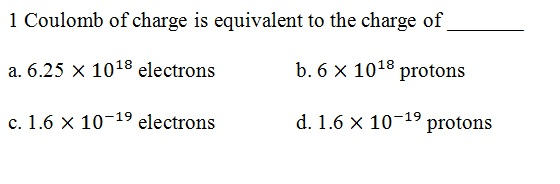A A B B C C D D
 Question 87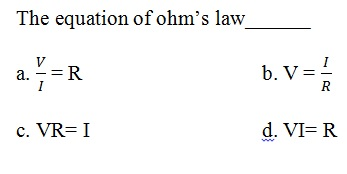A A B B C C D D
 Question 88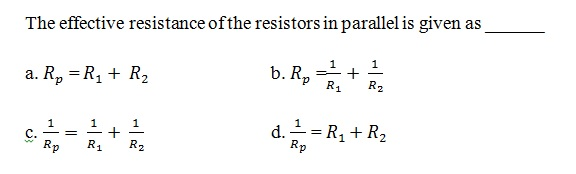A A B B C C D D
 Question 89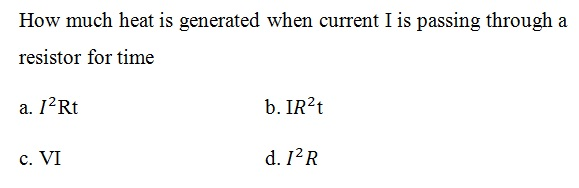A A B B C C D D
 Question 90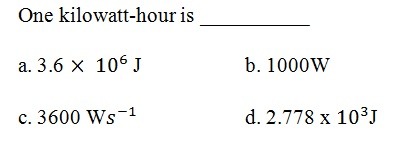A A B B C C D D
 Question 91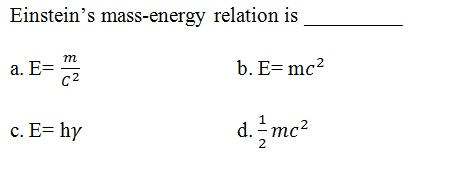A A B B C C D D
 Question 92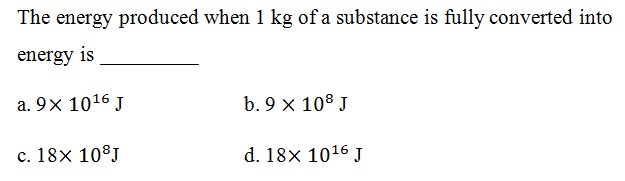A A B B C C D D
Once you are finished, click the button below. Any items you have not completed will be marked incorrect.
There are 92 questions to complete.